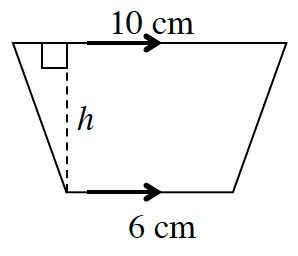### Home > CC4 > Chapter 5 > Lesson 5.3.1 > Problem5-89

5-89.

The area of the trapezoid at right is $56\text{ cm}^2$. What is the height, $h$? (Hint: The formula for the area of a trapezoid can be written as $A = \frac { 1 } { 2 }(b_1 + b_2)h$, where $b_1$ and $b_2$ are the lengths of the bases.) Show all work. Homework Help ✎What is the formula for the area of a trapezoid?
Use that to solve for $h$.

$7$ cm